# Obtuse Angle

Go back to  'Angles'

 1 Introduction To Angles 2 What is an Obtuse Angle? 3 Examples of Obtuse Angles 4 Obtuse Angle of a Triangle 5 Obtuse Angles of a Rhombus 6 Obtuse Angles of a Parallelogram 7 Solved Examples on Obtuse Angles 8 Thinking Out of the box! 9 Practice Questions on Obtuse Angles 10 Important Notes on Obtuse Angles 11 Maths Olympiad Sample Papers 12 Frequently Asked Questions (FAQs)

We at Cuemath believe that Math is a life skill. Our Math Experts focus on the “Why” behind the “What.” Students can explore from a huge range of interactive worksheets, visuals, simulations, practice tests, and more to understand a concept in depth.

Book a FREE trial class today! and experience Cuemath's LIVE Online Class with your child.

## Introduction To Angles

When two rays meet at a vertex, an angle is formed.

An angle is represented by the symbol $$\angle$$ and is measured in degrees $$^\circ$$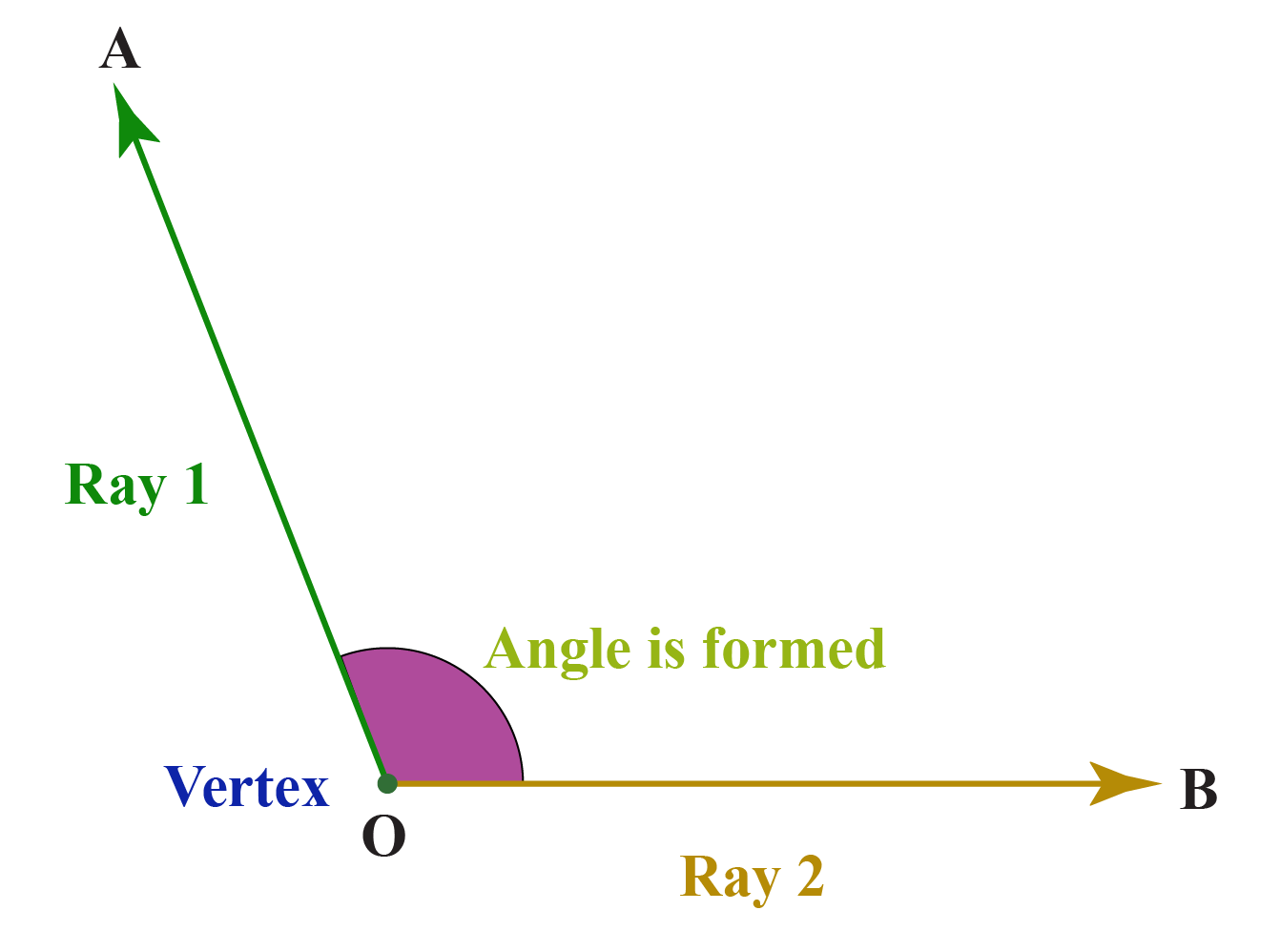## What is an Obtuse Angle?

The definition of an obtuse angle in Geometry states that an angle whose measure is greater than $${90^\circ}$$ and less than $${180^\circ}$$ is called an obtuse angle.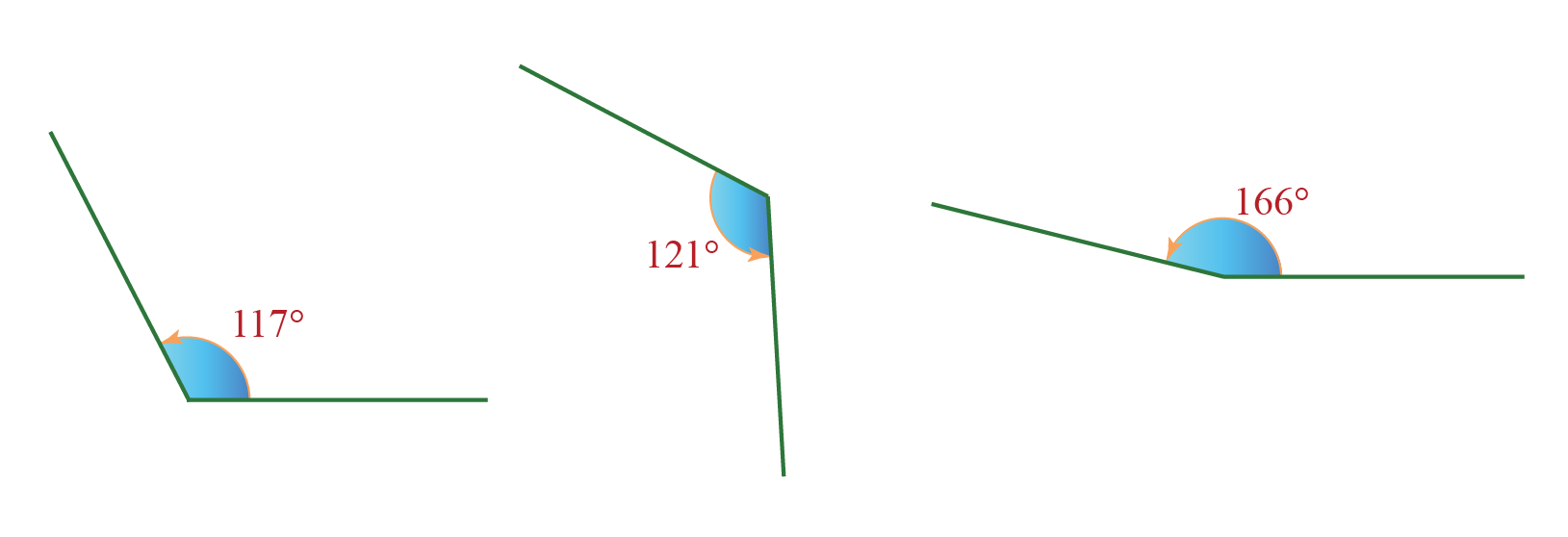Here is an activity for you to try.

Drag the point B to the left and observe all the obtuse angle measures.

## Examples of Obtuse Angles

Let's recall the definition of obtuse angles in Math.

We know that angles measuring greater than $$90^\circ$$ and less than $$180^\circ$$ are called obtuse angles.

Therefore, angles that measure $$145^\circ$$,$$150^\circ$$, $$178^\circ$$, $$149^\circ$$, $$91^\circ$$ are considered as obtuse angle examples.

Here are some real-life examples of obtuse angles.

Can you observe the obtuse angles in all these images?

Can you think of more objects in real life that include obtuse angles?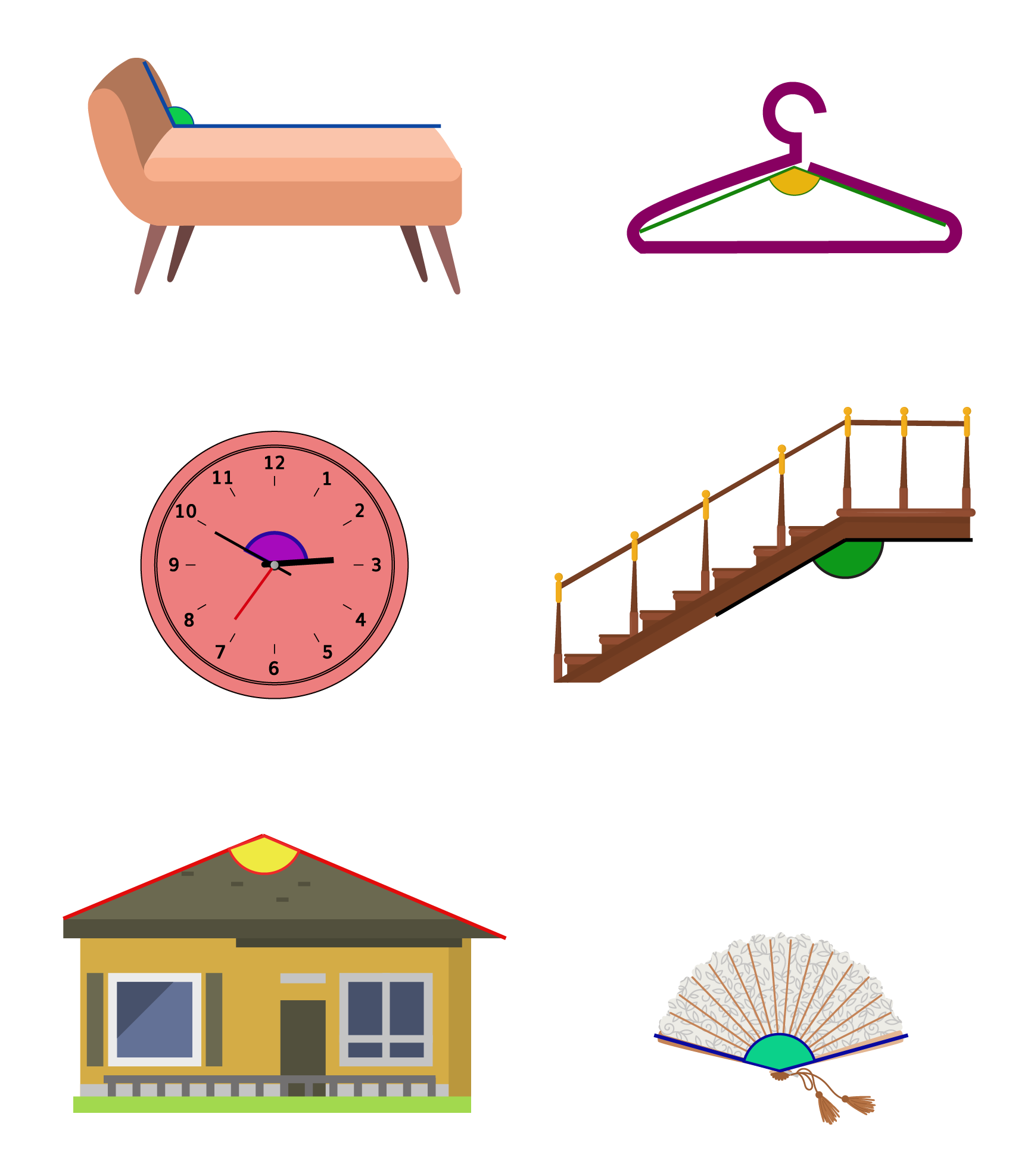## Obtuse Angle of a Triangle

When one of the vertex angles of a triangle is greater than $$90^\circ$$, it is called an obtuse triangle.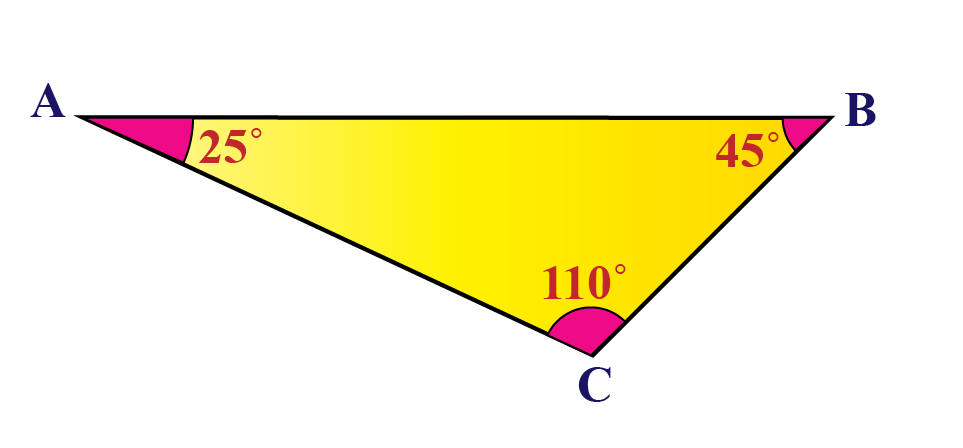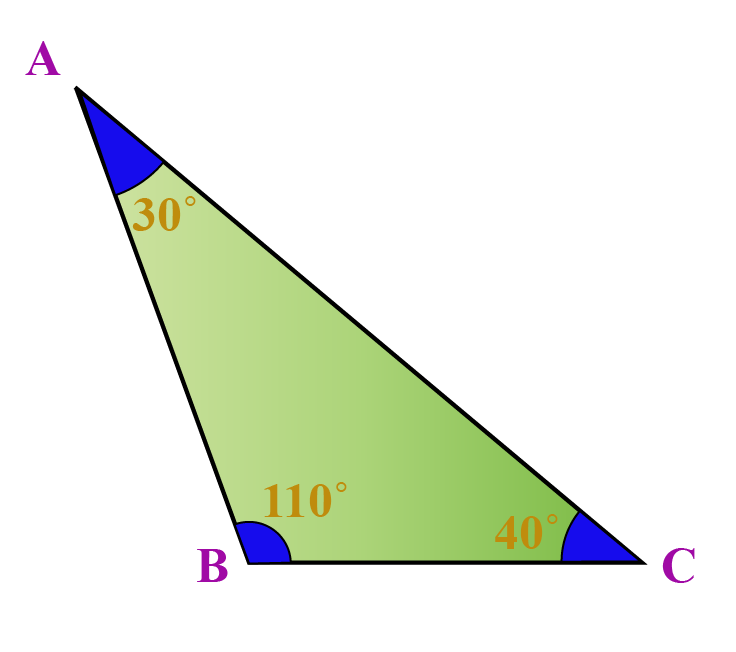The triangles above have one angle greater than $$90^\circ$$

Hence, they are called obtuse-angled triangles or simply obtuse triangles.

In an obtuse triangle, the sum of the squares of the two sides is less than the square of the longest side.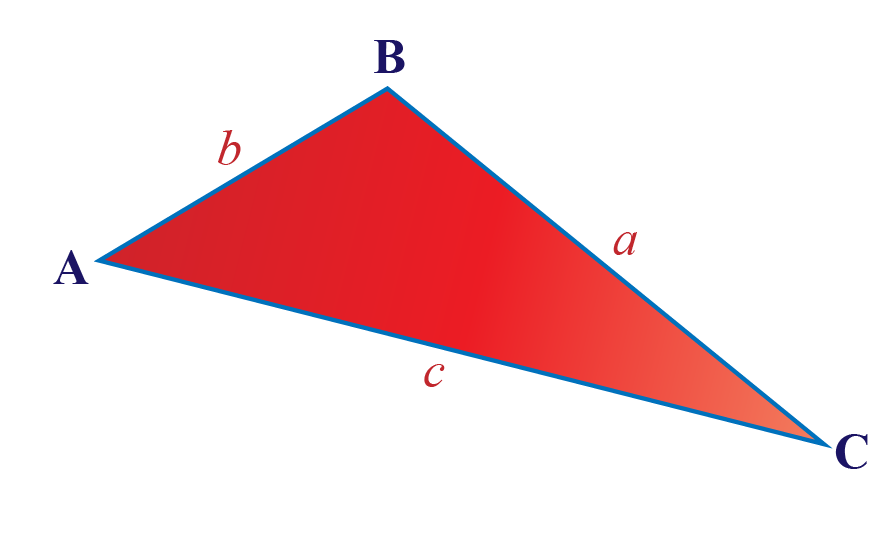In $$\Delta ABC$$, the sides measure $$a,b,c$$ such that $$c$$ is the largest side, thus:

 $$a^2 + b^2 < c^2$$

Conversely, if in a triangle, if $$a^2 + b^2 < c^2$$, then the triangle is an obtuse triangle.

## Obtuse Angles of a Rhombus

A rhombus is a special type of quadrilateral which includes:

• four equal sides
• two pairs of parallel sides
• equal opposite angles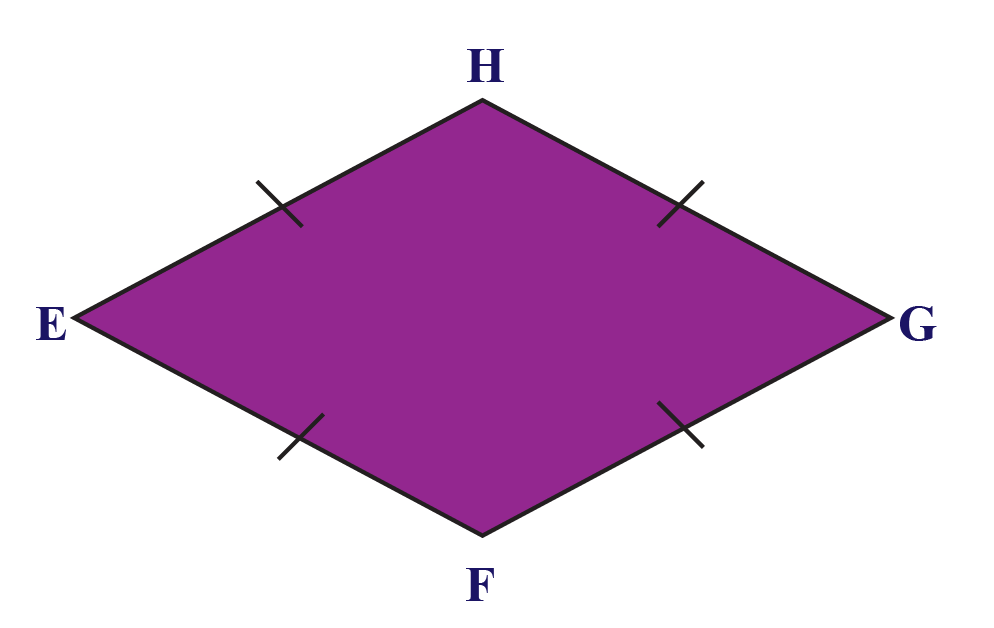The sum of the interior angles of any quadrilateral is $$180^\circ$$ and in a rhombus, consecutive angles are supplementary and opposite angles are equal.

Thus, at any given time, a  rhombus has two obtuse angles that are equal and the other two angles are acute and they are also  equal.

## Obtuse Angles of a Parallelogram

A Parallelogram is a special type of quadrilateral which includes:

• two pairs of parallel sides
• opposite sides of equal lengths
• equal opposite angles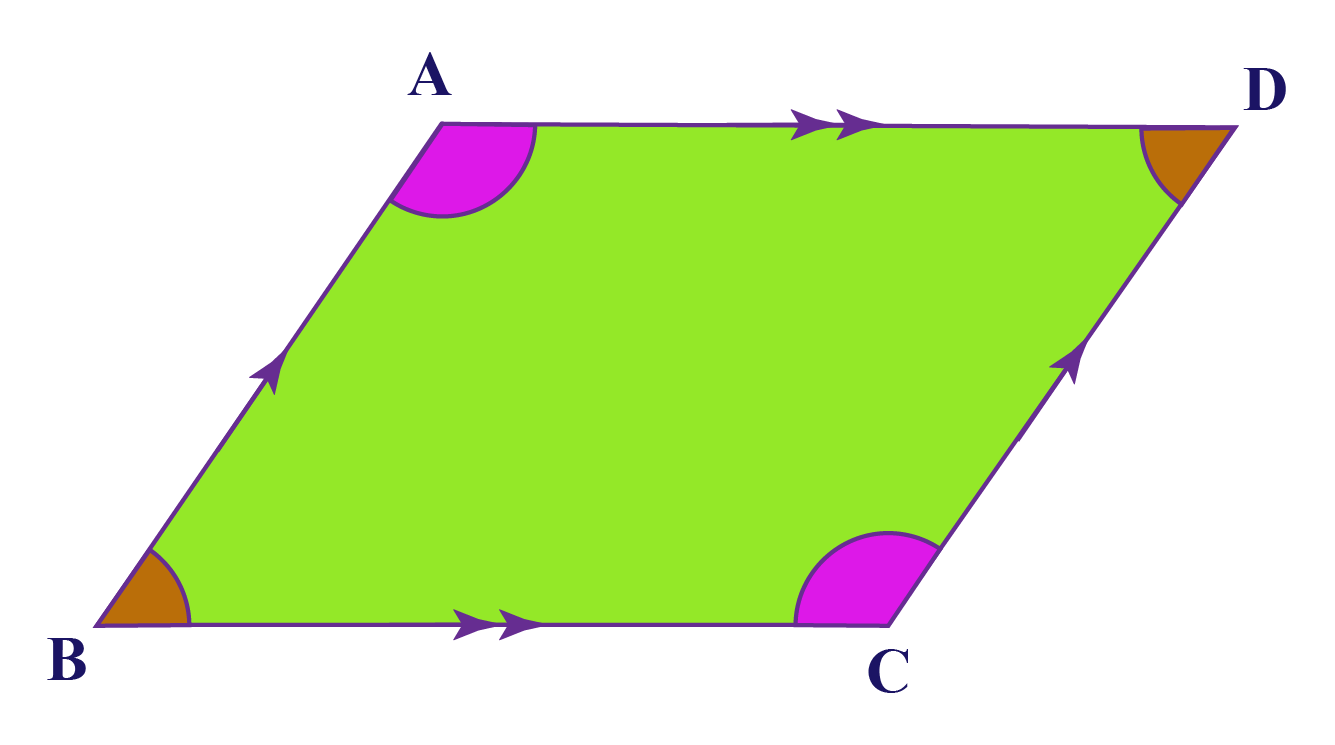The sum of the interior angles of any quadrilateral is $$180^\circ$$ and in a parallelogram, consecutive angles are supplementary and opposite angles are equal.

Thus, at any given time, a  parallelogram has two obtuse angles that are equal and the other two angles are acute and they are also  equal.Think Tank
1. Can a triangle have more than one obtuse angles?
2. Which polygon has all its internal angles as obtuse angles?
3. Can there be a parallelogram without an obtuse angle? If so, what shape can it be?

Help your child score higher with Cuemath’s proprietary FREE Diagnostic Test. Get access to detailed reports, customized learning plans, and a FREE counseling session. Attempt the test now.

## Solved Examples

 Example 1

Here is a small interactive activity; few random angles are generated.

Measure the angles using the protractor and identify the obtuse angles.

Solution:

All the angles measure more than $$90^\circ$$ and less than $$180^\circ$$

They are all obtuse angles.

 All the given angles are obtuse angles.
 Example 2

Choose all the obtuse angles from the following figures.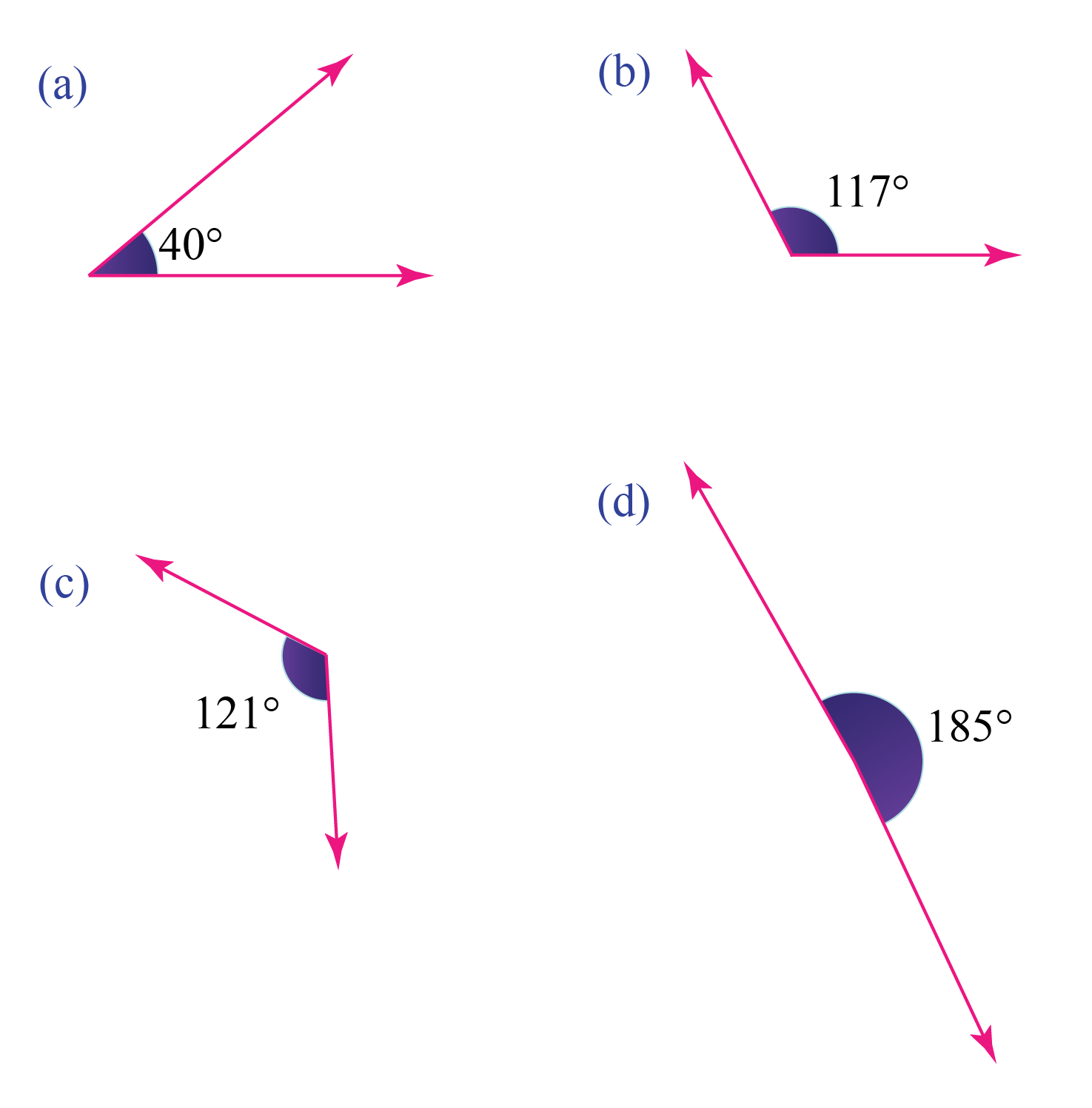Solution:

Option (b) and option (c) are more than $$90^\circ$$ and less than $$180^\circ$$

Hence, they are obtuse angles.

 Option (b) and option (c) are obtuse angles.
 Example 3

At what times, in the clocks shown below, an acute angle is formed?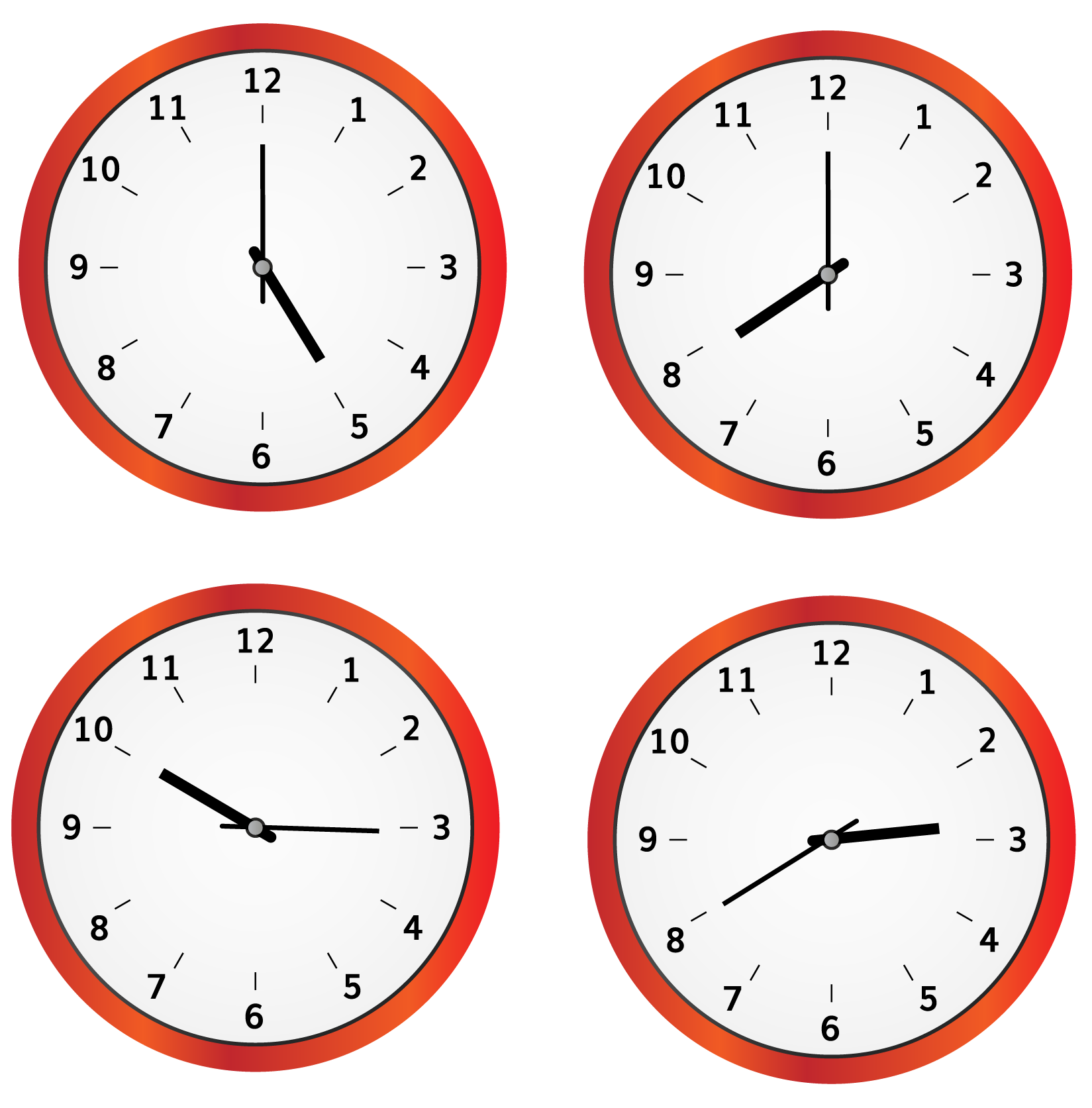Solution:

We can observe that in all instances, an obtuse angle is formed between the hour's hand and minutes hand of the clock.

 $$therefore$$ An obtuse angle is formed at 5:00, 10:15, 2:40 and 8:00.
 Example 4

Can an obtuse triangle have sides measuring 4 cm, 5 cm and 8 cm?

Solution:

In an obtuse triangle, the sum of the square of two sides should be less than the square of the greatest side.

i.e   $$a^2 + b^2 < c^2$$ where c is the largest side.

Let a = 4 cm

b = 5 cm

c = 8 cm (largest side)

$$a^2 = 16$$

$$b^2 = 25$$

$$c^2 = 64$$

$$a^2 +b^2= 16 +25 = 41$$

Since  $$41 < 64$$

$$\implies a^2 + b^2$$  is less than $$c^2$$

Hence, the given measures form an obtuse triangle.

 4 cm, 5 cm and 8 cm forms an obtuse triangle.
 Example 5

Which vertices of the parallelogram $$ABCD$$ have obtuse angles?

What is their measure?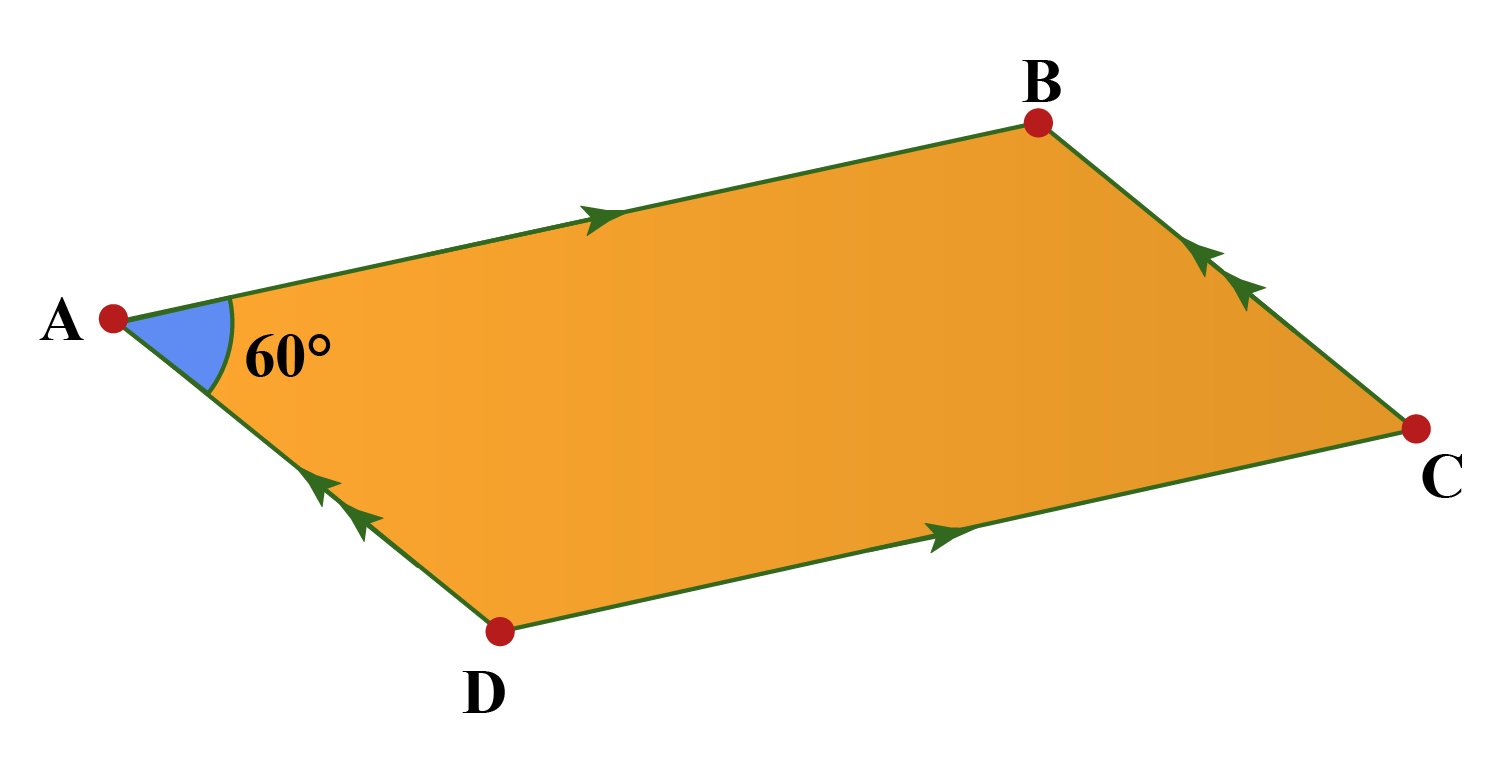Solution:

In a parallelogram, consecutive angles are supplementary and opposite angles are equal.

Since $$\angle A$$ = $$60^\circ$$, the opposite angle $$\angle C$$ is also $$60^\circ$$

$$\angle A$$ + $$\angle D$$ = $$180^\circ$$ (consecutive angles are supplementary)

Therefore, $$\angle D$$ =  $$120^\circ$$ and $$\angle B$$ = $$120^\circ$$ (opposite angles are equal)

 $$\angle B$$ and $$\angle D$$ are obtuse angles and they measure 120° each

Have a doubt that you want to clear? Get it clarified with simple solutions on Obtuse Angle from our Math Experts at Cuemath’s LIVE, Personalised and Interactive Online Classes.

Make your kid a Math Expert, Book a FREE trial class today!

## Practice Questions

Here are a few activities for you to practice.

IMO (International Maths Olympiad) is a competitive exam in Mathematics conducted annually for school students. It encourages children to develop their math solving skills from a competition perspective.

## 1. How do you create an obtuse angle?

The definition of an obtuse angle in Geometry states that an angle larger than $$90^\circ$$ but less than $$180^\circ$$ is called as an obtuse angle.

We can use a protractor and mark any angle between $$90^\circ$$ and $$180^\circ$$ to make an obtuse angle.

## 2. What are some examples of obtuse angles?

$$145^\circ$$,$$150^\circ$$, $$178^\circ$$, $$149^\circ$$, $$91^\circ$$ are all examples of obtuse angles as they are more than $$90^\circ$$ and less than $$180^\circ$$.

## 3. How do you determine an obtuse angle?

The definition of an obtuse angle states that if an angle measures more than $$90^\circ$$ and less than $$180^\circ$$, it is an obtuse angle.

We can use a protractor and verify the same.

More Important Topics
Numbers
Algebra
Geometry
Measurement
Money
Data
Trigonometry
Calculus